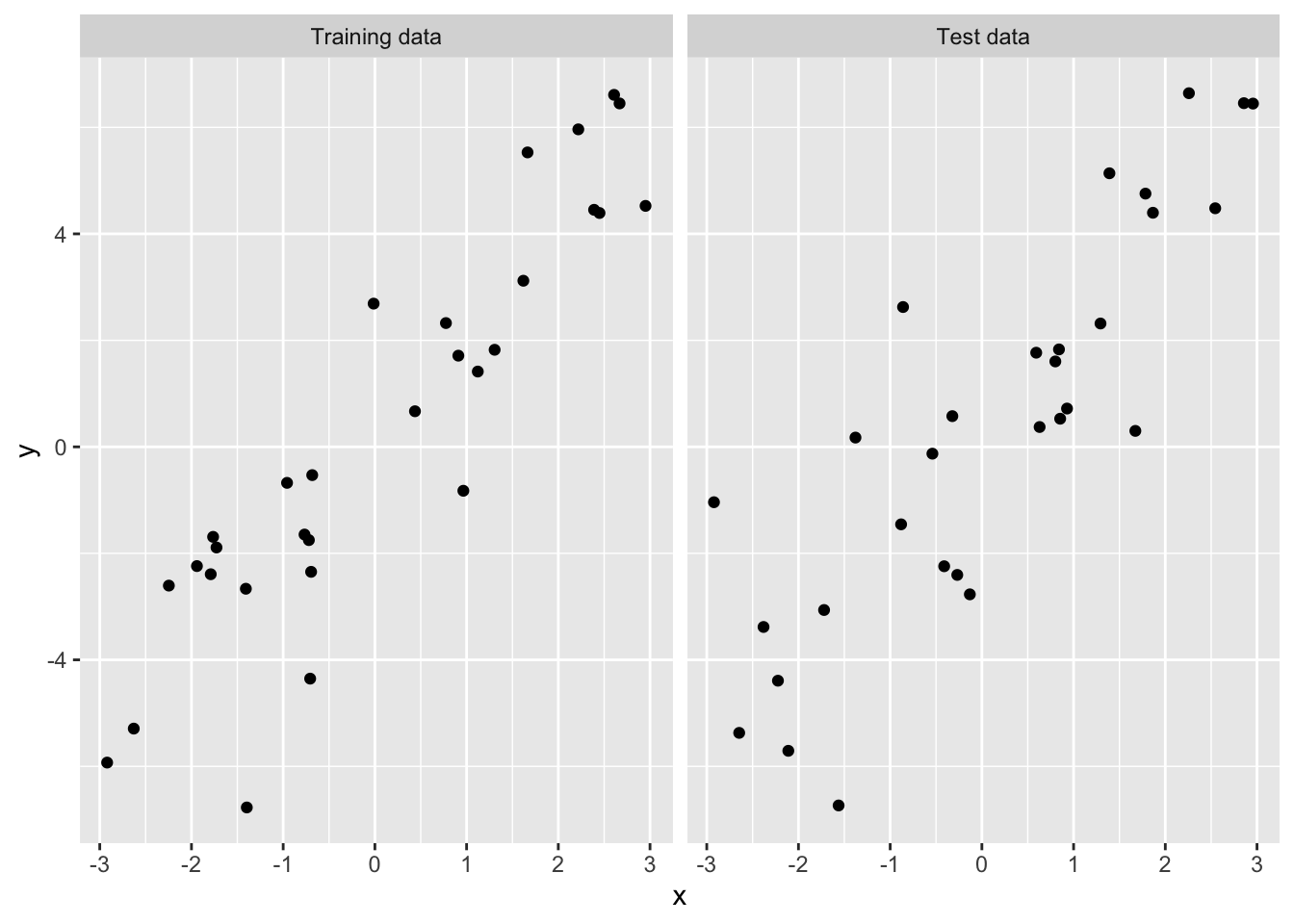Name:
Andrew ID:
Collaborated with:

This lab is to be done in class (completed outside of class if need be). You can collaborate with your classmates, but you must identify their names above, and you must submit your own lab as an knitted HTML file on Canvas, by Tuesday 10pm, this week.

This week’s agenda: understanding training and testing errors, implementing sample-splitting and cross-validation (optional), and trying a bunch of statistical prediction methods (also optional).

# Practice with training and test errors

The code below generates and plots training and test data from a simple univariate linear model, as in lecture. (You don’t need to do anything yet.)

library(tidyverse)
set.seed(1)
n <- 30
x <- sort(runif(n, -3, 3))
y <- 2*x + 2*rnorm(n)
x0 <- sort(runif(n, -3, 3))
y0 <- 2*x0 + 2*rnorm(n)

data_vis <- data.frame(x = c(x, x0),
y = c(y, y0),
tt = factor(rep(c("Training data", "Test data"),
each = n),
levels = c("Training data", "Test data")))

ggplot(data_vis) +
geom_point(aes(x = x, y = y)) +
facet_grid(~tt)• 1a. For every $$k$$ in between 1 and 15, regress the training data’s y onto a polynomial in it’s x of degree $$k$$. Hint: look at the lecture to see how to use the poly() function. Then use this fitted model to predict y0 from x0, and record the observed test error. Also record the observed training error. Plot the test error and training errors curves, as functions of $$k$$, on the same plot, with properly labeled axes, and an informative legend. What do you notice about the relative magnitudes of the training and test errors? What do you notice about the shapes of the two curves? If you were going to select a regression model based on training error, which would you choose? Based on test error, which would you choose?

• 1b. Without any programmatic implementation, answer: what would happen to the training error in the current example if we let the polynomial degree be as large as 29?

• 1c. Modify the above code for the generating current example data so that the underlying trend between y and x, and y0 and x0, is cubic (with a reasonable amount of added noise). Recompute training and test errors from regressions of y onto polynomials in x of degrees 1 up to 15. Answer the same questions as before, and notably: if you were going to select a regression model based on training error, which would you choose? Based on test error, which would you choose?

# Sample-splitting with the prostate cancer data

Below, we read in data on 97 men who have prostate cancer (from the book The Elements of Statistical Learning). (You don’t need to do anything yet.)

pros_df <- read.table(
"https://web.stanford.edu/~hastie/ElemStatLearn/datasets/prostate.data")
dim(pros_df)
##  97 10
head(pros_df)
##       lcavol  lweight age      lbph svi       lcp gleason pgg45       lpsa
## 1 -0.5798185 2.769459  50 -1.386294   0 -1.386294       6     0 -0.4307829
## 2 -0.9942523 3.319626  58 -1.386294   0 -1.386294       6     0 -0.1625189
## 3 -0.5108256 2.691243  74 -1.386294   0 -1.386294       7    20 -0.1625189
## 4 -1.2039728 3.282789  58 -1.386294   0 -1.386294       6     0 -0.1625189
## 5  0.7514161 3.432373  62 -1.386294   0 -1.386294       6     0  0.3715636
## 6 -1.0498221 3.228826  50 -1.386294   0 -1.386294       6     0  0.7654678
##   train
## 1  TRUE
## 2  TRUE
## 3  TRUE
## 4  TRUE
## 5  TRUE
## 6  TRUE
• 2a. As we can see, the designers of this data set already defined training and test sets for us, in the last column of pros_df! Split the prostate cancer data frame into two parts according to the last column, and report the number of observations in each part. On the training set, fit a linear model of lpsa on lcavol and lweight. On the test set, predict lpsa from the lcavol and lweight measurements. What is the test error?

• 2b. Using the same training and test set division as in the previous question, fit a linear model on the training set lpsa on age, gleason, and pgg45. Then on the test set, predict lpsa from the relevant predictor measurements. What is the test error?

• 2c. How do the test errors compare in the last two questions? Based on this comparison, what regression model would you recommend to your clinician friend? What other considerations might your clinician friend have when deciding between the two models that is not captured by your test error comparison?